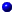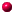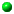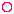• Maths index

## Binary Arithmetic

If I gave you a red ball and a blue ball and asked you to do arithmetic with them using one colour for one number and the other for another number (no other numbers allowed) I would be asking you to do binary arithmetic.

Binary is from the Latin binarius, which means doing it with two together (bini + ary)

At first sight, it may seem that you cannot count higher than two. But this is not so. In our normal counting we have only ten digits (0 to 9), but, by putting them in different places, we can carry on counting until we stop with exhaustion. We can do the same with two balls.

 The blue ball on its own is 0 The red ball on its own is 1 The red ball followed by the blue ball is 2 Two red balls is three The red ball followed by two blue balls is four The red ball, a blue ball, then a red ball is five Two red balls then a blue ball is six Three red balls is seven The red ball followed by three blue balls is eight The red ball, two blue balls, then a red ball is nine A red ball, a blue ball, a red ball, then a blue ball is ten A red ball, a blue ball, then two red balls is eleven Two red balls and two blue balls is twelve Two red balls, a blue ball, then a red ball is thirteen Three red balls, then a blue ball is fourteen Four red balls is fifteenWe can make Binary numbers by writing 0 for the blue ball and 1 for the red balls.

In binary numbers:

 0 means 0 1 means 1 10 means two 11 means three 100 means four 101 means five 110 means six 111 means seven 1000 means eight 1001 means nine 1010 means ten 1011 means eleven 1100 means twelve 1101 means thirteen 1110 means fourteen 1111 means fifteen There are 10 types of people in the world - Those who understand binary and Those who do not When you understand that joke you understand binary

Click here for an electronic calculator that converts our normal numbers to binary numbers

If all this driven you crazy, then pity the computer you are reading this on. Computers do all their counting in binary arithmetic. In fact, they do everything in binary. When you move the scroll bar the command is converted into binary patterns for the computer to understand it.

If you enjoy binary arithmetic and you can do it very very fast, perhaps you should apply for a job as a computer.Study LinkAndrew Roberts' web Study GuideTop of PageTake a Break - Read a PoemClick coloured words to go where you want